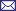401k: Should You Invest? An analysis of whether 401ks are still the great deal they always have been.FeedbackBy Preston Hunt, 01 March 2006

## Background

About a month ago, I blogged about Suze Orman's view on 401ks: She recommends against contributing to your 401k, beyond what is required to guarantee any employer matching.

After discussing it with some friends, I decided that an algebraic analysis was in order to see if this is good advice:

``````P = Starting principal
Tp = Present tax rate
Tf = Future tax rate
Y = Number of years before money is needed/withdrawn
R = Annual equity return
L = Future long-term capital gain rate + state income tax (if any)

Future value assuming continuous compounding = P * e^(n*R*T)``````

## Scenario 1 (401k)

Contribute tax-free P into 401k, let it grow for Y years, earning no dividends or capital gains. Withdraw and pay taxes Tf after Y years.

``FV1 = (P * e^(Y*R)) * (1 - Tf)``

## Scenario 2 (no 401k)

Pay taxes right away on P and put in regular taxable investment account for Y years, assuming no dividends or capital gains. Close account and withdraw after Y years, paying long term capital gains on the earnings since inception.

``````Starting equity, tax paid = E = P*(1-Tp)
Future value before taxes = Bt = (E * e^(Y*R))
Additional taxes owed = Ta = (Bt - E) * L
Final amount in pocket = Bt - Ta

After some algebra...
FV2 = P*(1-Tp) * ((1-L)*e^(Y*R) + L)``````

## Which one's better?

Set FV1 = FV2 and solve for the future tax rate Tf at which FV2 becomes more profitable.

That's some nasty algebra to solve... so remembering a technique that my finance friend Sam often uses, I punched all of this into Excel and used the numerical solver. Here's the source file: 401k analysis.xls.

Assuming P=10, Y=26, R=0.09, Tp=0.37, L=0.20, and solving for Tf, we get that Tf=0.48. So, as long as the tax rate doesn't go up above 48%, then 401k would still be a better investment.

And this analysis disregarded the effect of taxes on intermediate dividens and capital gains, which would make the 401k more attractive. On the other hand, this analysis didn't take into account the effect of 401k management fees, which could easily swing the balance back the other way.